# Study of Natural Convection Inside Inclined Nanofluid Cavity with Hot Inner Bodies (Circular and Ellipse Cylinders)

Study of Natural Convection Inside Inclined Nanofluid Cavity with Hot Inner Bodies (Circular and Ellipse Cylinders)

Makki Noori Jawad Ibrahim Karrar A. Hammoodi| Ahmed Dhafer Abdulsahib Mujtaba A. Flayyih

MSC Mechanical Engineering, Ministry of Education, General Directorate of Vocational Education, Babylon 51001, Iraq

Refrigeration & Air-Conditioning Technologies Department, College of Engineering, University of Warith Al-Anbiyaa, Karbala 56001, Iraq

Ministry of Electricity, General Company of Electricity AL-Furat Middle Region, East Diwanyah Diesel Power Plant, Diwanyah 58001, Iraq

Biomedical Engineering Department, Al-Mustaqbal University College, Babylon 51001, Iraq

Corresponding Author Email:
karrar.al@uowa.edu.iq
Page:
699-705
|
DOI:
https://doi.org/10.18280/ijht.400306
13 March 2022
|
Accepted:
12 June 2022
|
Published:
30 June 2022
| Citation

OPEN ACCESS

Abstract:

Due to its engineering uses in recent years, natural convection within cavities has been studied to enhance heat transfer in various package shapes by infusing the base fluid with nanoparticles. In this paper, we examined natural convection in a square cavity with an inclined roof of Ag–water nanofluid and internal bodies (circular and elliptical cylinders) at the enclosure's center. The (top, bottom, and circular cylinder) walls are assumed to be adiabatic, whereas the (ellipse cylinders and left sidewall) are warmed and the right sidewall is maintained cool. The COMSOL program is based on the "Galerkin finite element approach" in terms of numerical computations. The "Rayleigh number" (Ra) (103–106) is utilized, as is the solid volume fraction (0.05), the angle of inclination (-45°, -30°, 0°, 30°, 45°), "the inner circular cylinder radius considered as (R=0.15)" and "the radius of the inner ellipse cylinder as (Rx=0.2 & Ry=0.15). At a high "Rayleigh number", the stream function has the lowest value when the caustic angle is tilted to (-30°). While it comes in second place, the angle of inclination (0°) gets the highest value. While at a low "Rayleigh number", there is no effect of the angle of the stream function. There was also a convergence in Nusselt numbers at any angle at the hot left wall, at 30° and 45°, and in the hot ellipse, at 60° and 90°, so we looked at them all.

Keywords:

nanofluid, natural convection, circular and ellipse cylinders, inclined square enclosure

1. Introduction

Because of the numerous applications such as solar energy collection, natural convection inside enclosures has been and continues to be an important subject of study [1-3].

The study of heat convection inside the cavity has advanced in recent years . Some researchers have resorted to enhancing heat transfer in enclosures when adding nanoparticles to the basic fluid .

The effect of the different parameters in a four-sided cavity occupied with nanofluid was examined by various researchers. Ho et al.  explained the influence of thermal conductivity on heat transfer. The free convection [7-9] is influenced by the inclination of the cavity. Sheikholeslami and Ellahi  deliberated a cubical enclosure heated, the outcomes revealed a "Nusselt number" that raises as (Ra) and volume fraction rises. Sheremet et al.  studied the generation of entropy using nanofluid. Emami et al. studied the impact of the cavity's inclination angle . A few researchers have studied the use of more than one cylinder inside the cavity.

Studies have taken another way to entry mixed convection limits by moving one or more of the walls of the enclosures to enhance heat transfer with different parameters [13-17]. Other studies examined mixed convection in another way, using an inner cylinder that rotates in both directions [18-23]. Mixed convection was studied using a rotating circular cylinder by Abdulsahib and Al-Farhany . Cylinder rotation is more pronounced when using the lower Rayleigh numbers.

Several studies have been conducted on the lid-driven cavity and cylinder rotation within it for their significant influence on mixed convection [25-30].

From the literature review above, the previous studies are summarized to clarify the position of the current study: Enhancing heat transfer in enclosures when adding nanoparticles to the basic fluid, the free convection is influenced by the inclination of the cavity, studies on the use of more than one cylinder inside the cavity, and studies on the lid-driven cavity and cylinder rotation and their influence on mixed convection.

From previous studies, it is clear that internal bodies inside an inclined cavity have not been studied for their effect on heat transfer [31, 32]. So, the present study looked at the impact of internal bodies (circular and elliptical cylinders) at the enclosure's center on natural convection inside inclined cavities with nanofluids by using different parameters like Rayleigh number, the angle of inclination, and solid volume fraction and studying its effect on streamline, isotherm lines, and Nusselt number. Figure 1 shows the flowchart of this research paper from the introduction with the research objective, methodology, boundary conditions of the enclosure, validation of the program, results and discussion, and conclusion.

## 1.png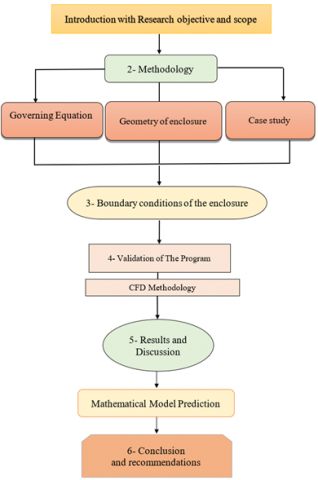Figure 1. Flowchart for present work

2. Methodology

Figure 2 shows a 2-D natural convection study in an inclined enclosure with a side length (L) and internal bodies (circular and ellipse cylinders). On the left side of the enclosure, the inner circular radius of cylinder is (R=0.15) and the inner ellipse cylinder radius is (Rx=0.2 & Ry=0.15). The container is filled with nanofluid. Water is used in the preparation of nanofluid, and nanoparticles are added (Ag). Table 1 depicts thermophysical characteristics of a substance (water and Ag nanoparticles). The vertical left sidewall and elliptical cylinders are fixed at a constant temperature (Th), whereas the vertical right sidewall is maintained at a cool temperature (T) (Tc). Thermal isolation is provided by the horizontal walls and the round cylinder. The circular and ellipse cylinders are in the middle of an inclined cavity with several different angles (-45°, -30°, 0°, 30°, 45°). In this study, the effect of the above states on the isotherm's lines, stream function, and the local and average Nu is studied.

Table 1. Thermophysical characteristics of pure water and nanoparticles 

 Substance Water Ag $\rho$ (kg/m3) 997.1 10,500 Cp(J/kg.K) 4179 235 K (W/m.K) 0.613 429 β [1/T] 2.1*10-4 5.4*10-5

## 2.pngFigure 2. The problem's illustrative geometry

In this investigation, the following dimensional governing equations are considered [34, 35]:

Continuity equation:

$\frac{\partial {{U}_{nf}}}{\partial X}+\frac{\partial {{V}_{nf}}}{\partial Y}=0$      (1)

Momentum equation:

${{U}_{nf}}\frac{\partial {{U}_{nf}}}{\partial X}+{{V}_{nf}}\frac{\partial {{U}_{nf}}}{\partial Y}=-\frac{\partial P}{\partial X}+\frac{pr}{{{(1-\Phi )}^{2.5}}}\frac{{{\rho }_{f}}}{{{\rho }_{nf}}}\left( \frac{{{\partial }^{2}}{{U}_{nf}}}{\partial {{X}^{2}}}+\frac{{{\partial }^{2}}{{U}_{nf}}}{\partial {{Y}^{2}}} \right)$     (2)

${{U}_{nf}}\frac{\partial {{V}_{nf}}}{\partial X}+{{V}_{nf}}\frac{\partial {{V}_{nf}}}{\partial Y}=-\frac{\partial P}{\partial Y}+\frac{pr}{{{(1-\Phi )}^{2.5}}}\frac{{{\rho }_{f}}}{{{\rho }_{nf}}}\left( \frac{{{\partial }^{2}}{{V}_{nf}}}{\partial {{X}^{2}}}+\frac{{{\partial }^{2}}{{V}_{nf}}}{\partial {{Y}^{2}}} \right)+\frac{{{(\rho \beta )}_{nf}}}{{{\rho }_{nf\,}}{{\beta }_{f}}}Ra\,\Pr \,\theta$     (3)

Energy equation:

${{U}_{nf}}\frac{\partial {{\theta }_{nf}}}{\partial X}+{{V}_{nf}}\frac{\partial {{\theta }_{nf}}}{\partial Y}=\frac{{{\alpha }_{nf}}}{{{\alpha }_{f}}}\left( \frac{{{\partial }^{2}}{{\theta }_{nf}}}{\partial {{X}^{2}}}+\frac{{{\partial }^{2}}{{\theta }_{nf}}}{\partial {{Y}^{2}}} \right)$      (4)

The properties of nanofluid are calculated from the considered papers [34, 35].

${{\rho }_{nf}}=(1-\phi ){{\rho }_{f}}+\phi {{\rho }_{np}}$      (5)

${{(\rho \beta )}_{nf}}=(1-\phi ){{(\rho \beta )}_{f}}+\phi {{(\rho \beta )}_{np}}$      (6)

${{(\rho {{C}_{P}})}_{nf}}=(1-\phi ){{(\rho {{C}_{P}})}_{f}}+\phi {{(\rho {{C}_{P}})}_{np}}$      (7)

${{\alpha }_{nf}}=\frac{{{K}_{nf}}}{{{(\rho {{C}_{P}})}_{nf}}}$      (8)

${{\mu }_{nf}}=\frac{{{\mu }_{f}}}{{{(1-\phi )}^{2.5}}}$      (9)

${{K}_{nf}}={{K}_{f}}\frac{({{K}_{np}}+2{{K}_{f}})-2\phi ({{K}_{f}}-{{K}_{np}})}{({{K}_{np}}+2{{K}_{f}})+\phi ({{K}_{f}}-{{K}_{np}})}$      (10)

The following local "Nusselt numbers (NuL)" are employed alongside the vertical hot-wall on the enclosure's left side, as shown in papers 34 and 35, respectively:

$N{{u}_{L}}=-\left( \frac{{{K}_{nf}}}{{{K}_{f}}} \right)\,\frac{\partial T}{\partial X}$      (11)

3. Boundary Conditions of the Enclosure

The enclosure's boundary conditions are summarized as follows:

• The left and right walls of the cavity are:

${{\theta }_{nf}}=1,\,\,\,\,\,\,\,{{\theta }_{nf}}=0$

• The upper and lower walls of the cavity are:

${\partial {{\theta }_{nf}}}/{\partial Y}\;=0,\,\,\,\,\,\,{\partial {{\theta }_{nf}}}/{\partial Y}\;=0$

• At the surface, cylinders:

${\partial {{\theta }_{nf}}}/{\partial r}\;=1$

• All wall velocities are equal to zero.
4. Solution Procedure and Validation

In the present paragraph, we illustrate the numerical results, which are based upon the powerful software, COMSOL, in terms of streamlines and angular velocities, with “Ra" and "Re," The accuracy of the project has been confirmed when compared with the results of Yoo  as shown in the figures. Where the comparison was made at Ra=106, Re=20, and Pr=0.7. In Figure 3, the streamline was compared, and the results were identical. In Figure 4, the comparison was made with angular velocities, and the results were also identical. Therefore, the current code was used to find the results in this research paper.

## 3.png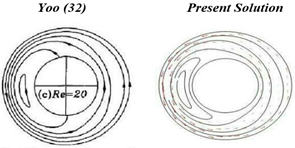Figure 3. Compare the streamlines of the current model to the results of Yoo  at (Ra=106, Re=20, and Pr=0.7)

## 4.png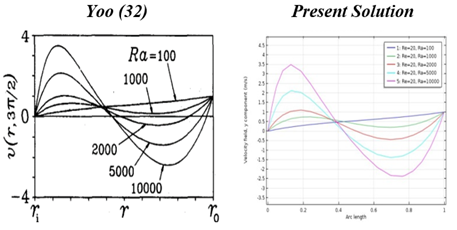Figure 4. Distribution comparison of the angular velocities as a function of “Ra” between the considered model and the results of Yoo  at when Re=20 with air fluid

5. Results

In the current work, the following parameters were used: "Rayleigh number" (Ra)" (103 up to 106) are used, the "solid volume fraction" (ϕ=0.05), inclination angle of (-45°, -30°, 0°, 30°, 45°), and the radius of the inner circular as (R=0.15) and the inner ellipse cylinder radius as (Rx=0.2 & Ry=0.15).

## 5.png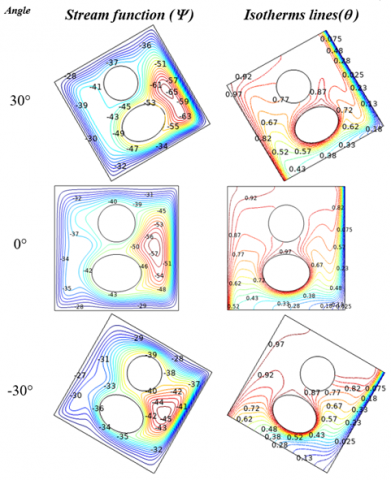Figure 5. Effect of cavity angle with adiabatic cylinder and heated elliptical on the stream function (left columns) and isotherm patterns (right columns) for (Ra=106, ϕ=0.05)

The current study aims to show the impact of the cavity's inclination angle on heat convection in the presence of internal bodies, cylinders, and an ellipse. Figure 5 illustrates the effect of the inclination angle (-30°, 0°, 30°) on the streamline and the isotherm lines at (Ra=106, =0.05). The left column shows the stream function. Noticed that the development of vortices on the right side of the ellipse. This is due to the ellipse's thermal impact. The fluid fracture is subjected to two effects, the first is the effect of the cold wall and the second is the effect of the heat from the (ellipse). The lowest value of the stream function when the caustic angle is tilted to (-30°) is (45). The reason here is the tilt of the angle, which is an obstacle to the movement of the fluid, so the effect of the cold wall is greater. The second place is the angle of inclination (0°), where it rises to (75), but at the angle of (30°) it gets the highest value of the stream action up to (65), and here the angle of inclination is important to simplify fluid movement and increase the effect of the hot wall and ellipse.

The right column represents the influence of heat transfer on the isotherm lines. It is worth observing that the heat transfer distribution within cavity is greatest at the hot left wall and the hot ellipse, whereas the heat transfer effect is almost non-existent behind the thermally insulated cylinder in front of the heat transfer from the left hot wall. Conclude from the figures that the distribution of heat transfer is best at an angle of inclination of 30°.

## 6.png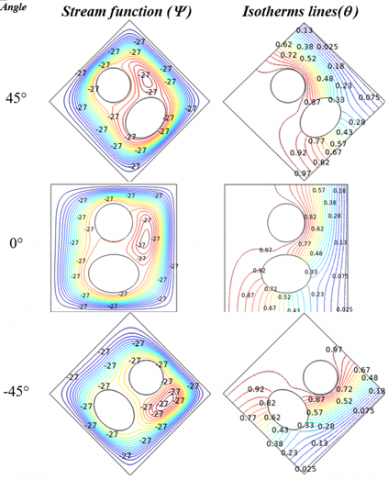Figure 6. Effect of cavity angle with heated cylinder and adiabatic elliptical on the stream function (left columns) and isotherm patterns (right columns) for (Ra=103, ϕ=0.05)

In Figure 6, different angles of inclination (-45°, 0°, 45°), and at a low "Rayleigh number" (103) were studied to find out the influence of the inclination angle in the presence of a hot inner body on heat transfer. It is found that the stream function values of the do not change clearly when changing the angle of inclination, but the difference is in the size of the vortices in addition to the change in their positions when changing from one angle to another, which indicates the impact of the inclination angle on the fluid movement mechanism, but with a low Rayleigh, the effect of the cold wall is higher and helps in that The presence of a thermally isolated cylinder From this, it can be said that the angle of inclination has no effect on the value of the stream function, so it has no effect. It only changes the shape and size of the vortices.

Also, the same physical interpretation for heat transfer lines, where the values of the steps and their order are the same when moving from one angle of inclination to another.

When comparing Figure 5 with Figure 6, it is noticed in general, regardless of the inclination angle, that the value of the streamline in Figure 5 is greater, in addition to the temperature distribution being more widespread, and the reason is the Rayleigh number 106 for Figure 5, while Figure 6 is studied at Rayleigh number 105. Different angles were also used in the two figures. When comparing, it is noted that the best case that can be obtained, which is the angle of 30° in Figure 5, where the value of the stream line reaches 65, as can be seen on the right side of the cavity, and the distribution of temperatures inside the cavities is more widespread.

## 7.png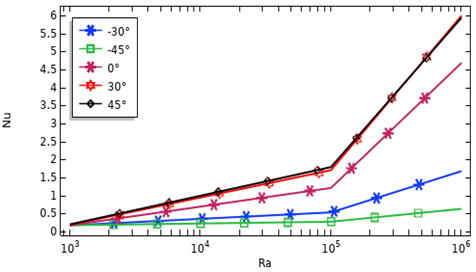Figure 7. Variation in the averaged "Nusselt number" on the cavity hot wall for various cavity angle using an adiabatic cylinder and a heated ellipse (ϕ=0.05)

Then the "Nusselt number" is an important guide of the heat transfer rate, we illustrate its inclination angle at low and high Ra along the hot wall and the elliptical cylinder as shown in Figures 7-8, respectively. It can be seen that, as in Figure 7, when the enclosure rotates in the negative direction and increases from 0° to 30° to 45°, there is a clear reduction in the average Nusselt number. This rise is becoming more noticeable as the rate of the "Rayleigh number" increases. However, there will be more enhancement in the "Nusselt number" as the angle of inclination increases from 0° to 30°. Additionally, the influence of the angle of inclination is negligible when it increases more than 45 degrees. When the angle of inclination is specifically increased in the positive direction by 30 degrees, the position of the cavity will be helpful to the movement of the fluid, as it will be freer. It is also noted that when the angle of inclination is increased to 45 degrees, there is no noticeable change in the value of the Nusselt number due to the small difference between the two angles, so the same physical effect will be had at any value of the Rayleigh number.

The highest value of the "Nusselt number" for the hot left wall is shown in Figure 8 at any "Rayleigh number" at an angle of between 30° and 45°, where the rotation of the cavity in a counter-clockwise direction gives a better situation for the fluid movement inside the cavity. As for the "Nusselt number," the ellipse, noticed a convergence in numbers, and the reason is that the ellipse is an internal body, so it has an angle of (0°), which gives the "Nusselt number" a higher approximate value. Additionally, as the Ra number will increases, the "Nusselt number" increases as well, which is a result of the increasing temperature, which increases the buoyancy force, resulting in increased heat transfer within the cavity.

## 8.png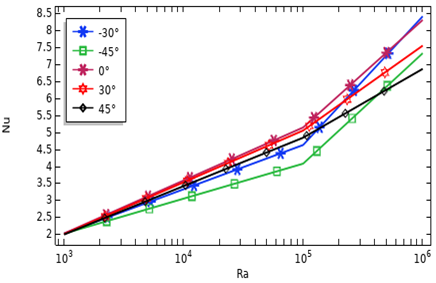Figure 8. Variation of the averaged "Nusselt number" on a heated elliptical wall for various values of the cavity angle using an adiabatic cylinder and a heated elliptical at (ϕ=0.05)

6. Conclusions

(1) Noticed the appearance of vortices on the right side of the ellipse. The reason is the effect of the heat of the ellipse on the fluid fracture. The first is the effect of the cold wall, and the second is the consequence of the heat from the ellipse.

(2) At the high "Rayleigh number," the lowest value of the stream function is when the caustic angle is tilted to (-30°). Its value is (45), while it comes in second place, the angle of inclination (0°), where it rises to (75), but at the angle of (30°) it gets the highest value of the stream function up to (65).

(3) For small values of Ra, the inclination angle has no effect on the stream function, and thus has no effect. Its effect is confined to modifying the shape and size of vortices only at the lowest of the Ra values.

(4) The highest Nu values for the hot left wall at any value of Ra at an angle of (30° and 45°) and as for the "Nusselt number" in the hot ellipse, noticed that convergence in Nusselt numbers at any angle.

(5) The limitations and future research direction as an extension of the current research: The effect of two thermal cylinders in different forms on heat transfer can be studied. A different nanofluid can also be used. Porous materials can be used with water or nanofluid.

Acknowledgment

Express gratitude to Al-Mustaqbal University College and University of Warith Al-Anbiyaa for their assistance and for allowing time to complete this research paper.

Nomenclature
 Symbol Description Cp Air heat capacity g Gravity acceleration k Thermal conductivity L Length of enclosure Nu Nusselt number as average value NuL Nusselt number as local value Pr Prandtl number R Cylinder radius Ra Rayleigh Number T Temperature U Velocity component in X-direction V Velocity component in Y-direction X Dimensionaless X-coordinates Y Dimensionaless Y-coordinates Greek symbols v Kinematic viscosity $\Psi$ Stream function as absolute value φ Solid volume fraction $\theta$ Dimensionless Temperature $\rho$ Density µ Dynamic viscosity α Thermal diffusivity β Thermal expansion coefficient Subscripts c Cold f Fluid (pure water) h Hot nf Nanofluid np Nanoparticle
References

 Mohebbi, R., Mehryan, S.A.M., Izadi, M., Mahian, O. (2019). Natural convection of hybrid nanofluids inside a partitioned porous cavity for application in solar power plants. Journal of Thermal Analysis and Calorimetry, 137(5): 1719-1733. https://doi.org/10.1007/s10973-019-08019-9

 Abu-Nada, E., Pop, I., Mahian, O. (2019). A dissipative particle dynamics two-component nanofluid heat transfer model: Application to natural convection. International Journal of Heat and Mass Transfer, 133: 1086-1098. https://doi.org/10.1016/j.ijheatmasstransfer.2018.12.151

 Zhang, R., Aghakhani, S., Pordanjani, A.H., Vahedi, S.M., Shahsavar, A., Afrand, M. (2020). Investigation of the entropy generation during natural convection of Newtonian and non-Newtonian fluids inside the L-shaped cavity subjected to magnetic field: Application of lattice Boltzmann method. The European Physical Journal Plus, 135(2): 184.

 de Vahl Davis, G. (1968). Laminar natural convection in an enclosed rectangular cavity. International Journal of Heat and Mass Transfer, 11(11): 1675-1693. https://doi.org/10.1016/0017-9310(68)90047-1

 Santra, A.K., Sen, S., Chakraborty, N. (2008). Study of heat transfer augmentation in a differentially heated square cavity using copper–water nanofluid. International Journal of Thermal Sciences, 47(9): 1113-1122. https://doi.org/10.1016/j.ijthermalsci.2007.10.005

 Ho, C.J., Chen, M.W., Li, Z.W. (2008). Numerical simulation of natural convection of nanofluid in a square enclosure: effects due to uncertainties of viscosity and thermal conductivity. International Journal of Heat and Mass Transfer, 51(17-18): 4506-4516. https://doi.org/10.1016/j.ijheatmasstransfer.2007.12.019

 Abu-Nada, E., Oztop, H.F. (2009). Effects of inclination angle on natural convection in enclosures filled with Cu–water nanofluid. International Journal of Heat and Fluid Flow, 30(4): 669-678. https://doi.org/10.1016/j.ijheatfluidflow.2009.02.001

 Ternik, P., Rudolf, R. (2012). Heat transfer enhancement for natural convection flow of water-based nanofluids in a square enclosure. International Journal of Simulation Modelling, 11(1): 29-39.

 Oztop, H.F., Mobedi, M., Abu-Nada, E., Pop, I. (2012). A heatline analysis of natural convection in a square inclined enclosure filled with a CuO nanofluid under non-uniform wall heating condition. International Journal of Heat and Mass Transfer, 55(19-20): 5076-5086. https://doi.org/10.1016/j.ijheatmasstransfer.2012.05.007

 Sheikholeslami, M., Ellahi, R. (2015). Three dimensional mesoscopic simulation of magnetic field effect on natural convection of nanofluid. International Journal of Heat and Mass Transfer, 89: 799-808. https://doi.org/10.1016/j.ijheatmasstransfer.2015.05.110

 Sheremet, M., Pop, I., Öztop, H.F., Abu-Hamdeh, N. (2017). Natural convection of nanofluid inside a wavy cavity with a non-uniform heating: Entropy generation analysis. International Journal of Numerical Methods for Heat & Fluid Flow.

 Emami, R.Y., Siavashi, M., Moghaddam, G.S. (2018). The effect of inclination angle and hot wall configuration on Cu-water nanofluid natural convection inside a porous square cavity. Advanced Powder Technology, 29(3): 519-536. https://doi.org/10.1016/j.apt.2017.10.027

 Gangawane, K.M., Oztop, H.F., Abu-Hamdeh, N. (2018). Mixed convection characteristic in a lid-driven cavity containing heated triangular block: Effect of location and size of block. International Journal of Heat and Mass Transfer, 124: 860-875. https://doi.org/10.1016/j.ijheatmasstransfer.2018.03.079

 Mahalakshmi, T., Nithyadevi, N., Oztop, H.F., Abu-Hamdeh, N. (2018). MHD mixed convective heat transfer in a lid-driven enclosure filled with Ag-water nanofluid with center heater. International Journal of Mechanical Sciences, 142: 407-419. https://doi.org/10.1016/j.ijmecsci.2018.05.008

 Yu, Q., Xu, H., Liao, S. (2018). Analysis of mixed convection flow in an inclined lid-driven enclosure with Buongiorno’s nanofluid model. International Journal of Heat and Mass Transfer, 126: 221-236. https://doi.org/10.1016/j.ijheatmasstransfer.2018.05.109

 Astanina, M.S., Sheremet, M.A., Oztop, H.F., Abu-Hamdeh, N. (2018). Mixed convection of Al2O3-water nanofluid in a lid-driven cavity having two porous layers. International Journal of Heat and Mass Transfer, 118: 527-537. https://doi.org/10.1016/j.ijheatmasstransfer.2017.11.018

 Abdulsahib, A.D., Al-Farhany, K. (2021). Review of the effects of stationary/rotating cylinder in a cavity on the convection heat transfer in porous media with/without nanofluid. Mathematical Modelling of Engineering Problems, 8(3): 356-364. https://doi.org/10.18280/mmep.080304

 Shih, Y.C., Cheng, Y.J. (2015). The effect of viscous dissipation on heat transfer in cavities of varying shape due to an inner rotating circular cylinder. Numerical Heat Transfer, Part A: Applications, 68(2): 150-173. https://doi.org/10.1080/10407782.2014.977134

 Selimefendigil, F., Ismael, M.A., Chamkha, A.J. (2017). Mixed convection in superposed nanofluid and porous layers in square enclosure with inner rotating cylinder. International Journal of Mechanical Sciences, 124: 95-108. https://doi.org/10.1016/j.ijmecsci.2017.03.007

 Selimefendigil, F., Öztop, H.F. (2018). Mixed convection of nanofluids in a three dimensional cavity with two adiabatic inner rotating cylinders. International Journal of Heat and Mass Transfer, 117: 331-343. https://doi.org/10.1016/j.ijheatmasstransfer.2017.09.116

 Hussein, A.K., Hamzah, H.K., Ali, F.H., Kolsi, L. (2020). Mixed convection in a trapezoidal enclosure filled with two layers of nanofluid and porous media with a rotating circular cylinder and a sinusoidal bottom wall. Journal of Thermal Analysis and Calorimetry, 141(5): 2061-2079. https://doi.org/10.1007/s10973-019-08963-6

 Ali, F.H., Hamzah, H.K., Abdulkadhim, A. (2019). Numerical study of mixed convection nanofluid in an annulus enclosure between outer rotating cylinder and inner corrugation cylinder. Heat Transfer—Asian Research, 48(1): 343-360. https://doi.org/10.1002/htj.21387

 Al-Farhany, K., Abdulsahib, A.D. (2021). Study of mixed convection in two layers of saturated porous medium and nanofluid with rotating circular cylinder. Progress in Nuclear Energy, 135: 103723. https://doi.org/10.1016/j.pnucene.2021.103723

 Abdulsahib, A.D., Al-Farhany, K. (2020). Numerical Investigation of the nanofluid mixed convection on two layers enclosure with rotating cylinder: High Darcy Number Effects. In IOP Conference Series: Materials Science and Engineering, 928(2): 022001. https://doi.org/10.1088/1757-899X/928/2/022001

 Abdulsahib, A.D., Hashim, A.S., Al-Farhany, K., Abdulkadhim, A., Mebarek-Oudina, F. (2021). Natural convection investigation under influence of internal bodies within a nanofluid-filled square cavity. Eur. Phys. J. Spec. Top. https://doi.org/10.1140/epjs/s11734-022-00584-9

 Barnoon, P., Toghraie, D., Dehkordi, R.B., Abed, H. (2019). MHD mixed convection and entropy generation in a lid-driven cavity with rotating cylinders filled by a nanofluid using two phase mixture model. Journal of Magnetism and Magnetic Materials, 483: 224-248. https://doi.org/10.1016/j.jmmm.2019.03.108

 Abdulsahi, A.D., Al-Farhany, K. (2020). Experimental investigation of mixed convection on a rotating circular cylinder in a cavity filled with nanofluid and porous media. Al-Qadisiyah Journal for Engineering Sciences, 13(2): 99-108.

 Abdulsahi, A.D., Al-Farhany, K. (2020). Experimental investigation of mixed convection on a rotating circular cylinder in a cavity filled with nanofluid and porous media. Al-Qadisiyah Journal for Engineering Sciences, 13(2): 99-108.

 Khalaf, A.F., Basem, A., Hussein, H.Q., Jasim, A.K., Hammoodi, K.A., Al-Tajer, A.M., Omer, I., Flayyih, M.A. (2022). Improvement of heat transfer by using porous media, nanofluid, and fins: A review. International Journal of Heat and Technology, 40(2): 497-521. https://doi.org/10.18280/ijht.400218

 Omar, I., Alrudhan, A., Hammoodi, K.A., Ali, A.M. (2022). Experimental and theoretical comparison between metallic and mirror reflectors with different receiver tank. Journal of Mechanical Engineering Research and Developments, 43(7): 51-61.

 Hammoodi, K.A., Hasan, H.A., Abed, M.H., Basem, A., Al-Tajer, A.M. (2022). Control of heat transfer in circular channels using oblique triangular ribs. Results in Engineering, 100471. https://doi.org/10.1016/j.rineng.2022.100471

 Mohammad, W.S., Murtadha, T.K., Ahmed, K.A. (2014). Using TermoDeck system for pre-cooling/heating to control the building inside conditions. Al-Khwarizmi Engineering Journal, 10(3): 13-24.

 Roslan, R., Saleh, H., Hashim, I. (2012). Effect of rotating cylinder on heat transfer in a square enclosure filled with nanofluids. International Journal of Heat and Mass Transfer, 55(23-24): 7247-7256. https://doi.org/10.1016/j.ijheatmasstransfer.2012.07.051

 Hussain, S.H., Rahomey, M.S. (2019). Comparison of natural convection around a circular cylinder with different geometries of cylinders inside a square enclosure filled with Ag-nanofluid superposed porous-nanofluid layers. Journal of Heat Transfer, 141(2): 022501. https://doi.org/10.1115/1.4039642

 Al-Srayyih, B.M., Gao, S., Hussain, S.H. (2019). Effects of linearly heated left wall on natural convection within a superposed cavity filled with composite nanofluid-porous layers. Advanced Powder Technology, 30(1): 55-72. https://doi.org/10.1016/j.apt.2018.10.007

 Yoo, J.S. (1998). Mixed convection of air between two horizontal concentric cylinders with a cooled rotating outer cylinder. International Journal of Heat and Mass Transfer, 41(2): 293-302. https://doi.org/10.1016/S0017-9310(97)00141-5